SSC CGL Previous Year Questions: Algebra- 3

# SSC CGL Previous Year Questions: Algebra- 3 - SSC CGL

Test Description

## 40 Questions MCQ Test SSC CGL (Tier - 1) - Previous Year Papers (Topic Wise) - SSC CGL Previous Year Questions: Algebra- 3

SSC CGL Previous Year Questions: Algebra- 3 for SSC CGL 2023 is part of SSC CGL (Tier - 1) - Previous Year Papers (Topic Wise) preparation. The SSC CGL Previous Year Questions: Algebra- 3 questions and answers have been prepared according to the SSC CGL exam syllabus.The SSC CGL Previous Year Questions: Algebra- 3 MCQs are made for SSC CGL 2023 Exam. Find important definitions, questions, notes, meanings, examples, exercises, MCQs and online tests for SSC CGL Previous Year Questions: Algebra- 3 below.
Solutions of SSC CGL Previous Year Questions: Algebra- 3 questions in English are available as part of our SSC CGL (Tier - 1) - Previous Year Papers (Topic Wise) for SSC CGL & SSC CGL Previous Year Questions: Algebra- 3 solutions in Hindi for SSC CGL (Tier - 1) - Previous Year Papers (Topic Wise) course. Download more important topics, notes, lectures and mock test series for SSC CGL Exam by signing up for free. Attempt SSC CGL Previous Year Questions: Algebra- 3 | 40 questions in 40 minutes | Mock test for SSC CGL preparation | Free important questions MCQ to study SSC CGL (Tier - 1) - Previous Year Papers (Topic Wise) for SSC CGL Exam | Download free PDF with solutions
 1 Crore+ students have signed up on EduRev. Have you?
SSC CGL Previous Year Questions: Algebra- 3 - Question 1

### Among the equations x + 2y + 9 = 0; 5x – 4 = 0; 2y – 13 = 0; 2x – 3y = 0, the equation of the straight line passing through origin is :       (SSC CGL 1st Sit. 2015)

Detailed Solution for SSC CGL Previous Year Questions: Algebra- 3 - Question 1

2x – 3y = 0 is passing through origion because its satisfy x = 0 and y = 0.

SSC CGL Previous Year Questions: Algebra- 3 - Question 2

### The area of the triangle formed by the graphs of the equations x = 0, 2x + 3y = 6 and x + y = 3 is       (SSC CGL 1st Sit. 2015)

Detailed Solution for SSC CGL Previous Year Questions: Algebra- 3 - Question 2

x = 0 ...(1)
2x + 3y = 6 ...(2)
x + y = 3 ...(3)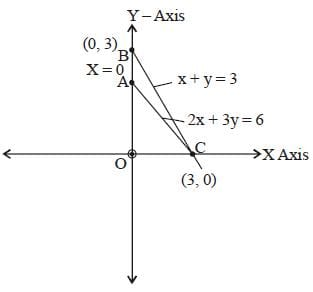2x + 3y = 6 ...(ii)
x = 0, y = 2
x = 3, y = 0
From eqn. (iii) x+ y = 3
x = 0, y = 3
x = 3, y = 0
Area made by these three lines
= Area of triangle OBC – Area of OAC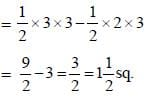SSC CGL Previous Year Questions: Algebra- 3 - Question 3

### If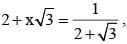then the simplest value of x is         (SSC CGL 1st Sit. 2015)

Detailed Solution for SSC CGL Previous Year Questions: Algebra- 3 - Question 3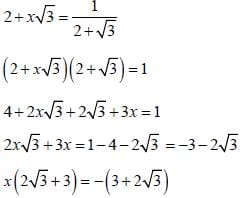x = – 1

SSC CGL Previous Year Questions: Algebra- 3 - Question 4

If x = 332, y = 333, z = 335, then the value of x3 + y3 + z3 – 3xyz is       (SSC CGL 1st Sit. 2015)

Detailed Solution for SSC CGL Previous Year Questions: Algebra- 3 - Question 4

Using the formula,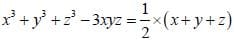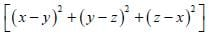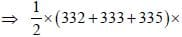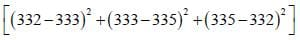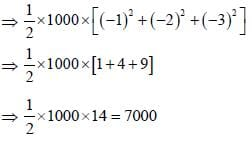SSC CGL Previous Year Questions: Algebra- 3 - Question 5

Ifthen the value of m is        (SSC CGL 1st Sit. 2015)

Detailed Solution for SSC CGL Previous Year Questions: Algebra- 3 - Question 5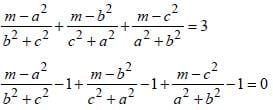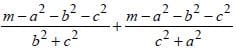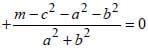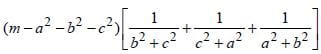= 0
m- a2 - b2 - c2 = 0
m = a2 + b2 + c2

SSC CGL Previous Year Questions: Algebra- 3 - Question 6

2x – ky + 7 = 0 and 6x – 12y + 15 = 0 has no solution for        (SSC CGL 1st Sit. 2015)

Detailed Solution for SSC CGL Previous Year Questions: Algebra- 3 - Question 6

For no solution, a = b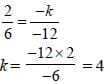SSC CGL Previous Year Questions: Algebra- 3 - Question 7

If m = –4, n = –2, then the value of m3 – 3m2 + 3m + 3n + 3n2 + n3 is       (SSC CGL 1st Sit. 2015)

Detailed Solution for SSC CGL Previous Year Questions: Algebra- 3 - Question 7

m3 – 3m2 + 3m + 3n + 3n2 + n3
⇒ (– 4)3 – 3 (– 4)2 + 3 (– 4) + 3 (– 2) + 3 (– 2)2 + (– 2)3
⇒ – 64 – 48 – 12 – 6 + 12 – 8
⇒ – 126

SSC CGL Previous Year Questions: Algebra- 3 - Question 8

The length of the portion of the straight line 3x + 4y = 12 intercepted between the axes is       (SSC CGL 1st Sit. 2015)

Detailed Solution for SSC CGL Previous Year Questions: Algebra- 3 - Question 8

Intercept can represent in the form of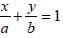To get x and y intercept, we have
3x + 4y = 12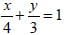So, triplets of 3, 4 and 5.
Hence, 5 is the length of portion of straight line.

SSC CGL Previous Year Questions: Algebra- 3 - Question 9

If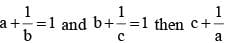is equal to        (SSC CHSL 2015)

Detailed Solution for SSC CGL Previous Year Questions: Algebra- 3 - Question 9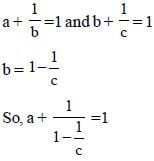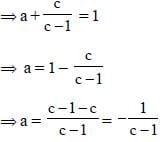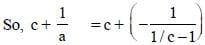= c– (c – 1)
= c – c + 1
= 1

SSC CGL Previous Year Questions: Algebra- 3 - Question 10

If (2a – 1)2 + (4b – 3)2 + (4c + 5)2 = 0 then the value of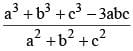is:        (SSC CHSL 2015)

Detailed Solution for SSC CGL Previous Year Questions: Algebra- 3 - Question 10

(2a – 1)2 + (4b – 3)2 + (4c + 5)2 = 0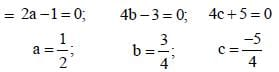a3 + b3 + c3 – 3abc = (a + b + c) (a2 + b2+ c2 – ab – bc – ca)
But a + b + c = 0
So, a3 + b3 +c3 – 3abc = 0
So,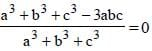SSC CGL Previous Year Questions: Algebra- 3 - Question 11

Two positive whole numbers are such that  the sum of the first and twice the second number is 8 and their difference is 2. The numbers are :        (SSC CHSL 2015)

Detailed Solution for SSC CGL Previous Year Questions: Algebra- 3 - Question 11

Let the two numbers are x and y
So, x + 2y = 8 … (i)
x – y = 2 … (ii)
Solving both equations
x = 4; y = 2
So, numbers are 4, 2.

SSC CGL Previous Year Questions: Algebra- 3 - Question 12

A number of boys raised ₹12,544 for a famine fund, each boy has given as many rupees as there were boys. The number of boys was:        (SSC CHSL 2015)

Detailed Solution for SSC CGL Previous Year Questions: Algebra- 3 - Question 12

Contribution of each boy = Number of boys
Total contribution raised = ₹ 12544
So, number of boys =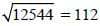SSC CGL Previous Year Questions: Algebra- 3 - Question 13

If for non–zero x, x2 – 4x – 1 = 0 the value of x2 - (1/x2) is :        (SSC CHSL 2015)

Detailed Solution for SSC CGL Previous Year Questions: Algebra- 3 - Question 13

x2 – 4x – 1 = 0 can be written as x2 – 4x + 4 – 1 = 4
So (x - 2)2 – 1 = 4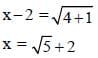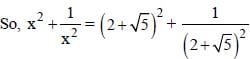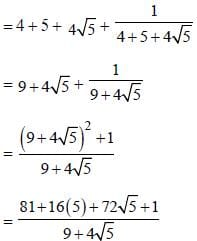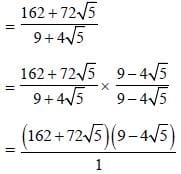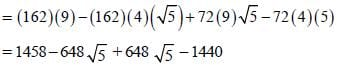= 1458 – 1440 = 18.

SSC CGL Previous Year Questions: Algebra- 3 - Question 14

If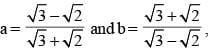then the value of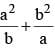is :        (SSC CHSL 2015)

Detailed Solution for SSC CGL Previous Year Questions: Algebra- 3 - Question 14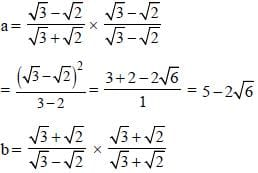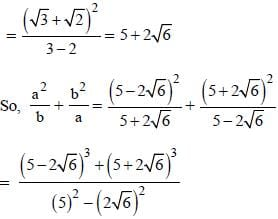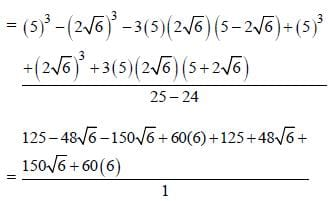= 125 + 125 + 360 + 360
= 250 + 720
= 970

SSC CGL Previous Year Questions: Algebra- 3 - Question 15

If p = –0.12, q = –0.01 & r = –0.015, then the correct relationship among the three is :        (SSC CHSL 2015)

Detailed Solution for SSC CGL Previous Year Questions: Algebra- 3 - Question 15

p = – 0.12 ; q = – 0.01; r = – 0.015
So, p < r ; p < q
= p < r < q

SSC CGL Previous Year Questions: Algebra- 3 - Question 16

If x + (1/x)= 2, then the value of x7 + (1/x5) is:      (SSC Sub. Ins. 2015)

Detailed Solution for SSC CGL Previous Year Questions: Algebra- 3 - Question 16

x + (1/x) = 2
Take cube on both sides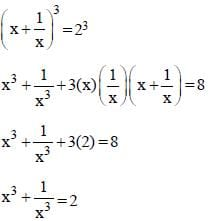Squaring on both sides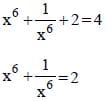Now multiplying both sides by x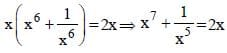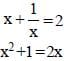x2 – 2x + 1 = 0
x2 – x – x +1 = 0
x(x – 1) –1(x – 1)
(x –1)2 = 0
x = 1
So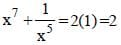SSC CGL Previous Year Questions: Algebra- 3 - Question 17

The term that should be added to (4x2 + 8x) so that resulting expression be a perfect square is:       (SSC Sub. Ins. 2015)

Detailed Solution for SSC CGL Previous Year Questions: Algebra- 3 - Question 17

4x2 + 8x = (2x)2 + 2(2x) (2) + (2)2
= (2x + 2)2 ∴ x2 + y2 + 2xy = (x + y)2
So, 4 should be added to make if perfect square.

SSC CGL Previous Year Questions: Algebra- 3 - Question 18

If 999x  + 888y = 1332
888x  + 999y = 555 then the value of x+y is:       (SSC Sub. Ins. 2015)

Detailed Solution for SSC CGL Previous Year Questions: Algebra- 3 - Question 18

999x + 888y = 1332
111(9x + 8y) = 1332
9x + 8y = 1332/111 = 12
9x + 8y = 12 ...(i)
888x + 999y = 555
8x + 9y = 5 ...(ii)
Solving (i) and (ii)
x = 4, y = – 3
x + y = 4 – 3 = 1

SSC CGL Previous Year Questions: Algebra- 3 - Question 19

If  32x -y = 3x +y = √27 , then the value of  3x -y will be :        (SSC Sub. Ins. 2015)

Detailed Solution for SSC CGL Previous Year Questions: Algebra- 3 - Question 19

32x – y = 3x + y = √27 = 33/2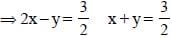4x – 2y = 3 ...(i)
2x + 2y = 3 ...(ii)
Solving equation (i) and (ii)
x = 1 y = 1/2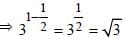SSC CGL Previous Year Questions: Algebra- 3 - Question 20

The mean of x and 1/x is M. Then the mean of x2 and 1/x2 is.      (SSC Sub. Ins. 2015)

Detailed Solution for SSC CGL Previous Year Questions: Algebra- 3 - Question 20

Mean of x and (1/x) = M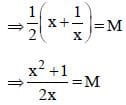Mean of x2 and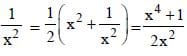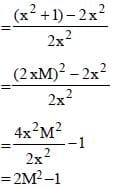SSC CGL Previous Year Questions: Algebra- 3 - Question 21

If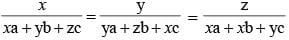and x + y + z ≠ 0, then each ratio is        (SSC CHSL 2014)

Detailed Solution for SSC CGL Previous Year Questions: Algebra- 3 - Question 21

Given,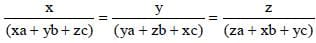We know that property of ratio,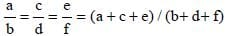So,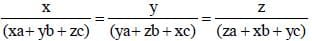= (x + y + z)/(xa + xb + xc + ya + yb + yc + za + zb + zc)
⇒ x / (xa + yb + zc) = y / (ya + zb + xc) = z / (za + xb + yc)
= x / (xa + yb + zc) = y (ya + zb + xc) = z / (za + xb + yc)
= (x + y + z) / {x(a + b + c) + y(a + b + c) + z(a + b + c)}
⇒ x(xa + yb + zc) = y(ya + zb + xc) = z(za + xb + yc)
= (x + y + z) / {(a + b + c) (x + y + z)
⇒ x / (xa + yb + zc) = y / (ya + zb + xc) = z / (za + xb + yc)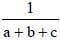SSC CGL Previous Year Questions: Algebra- 3 - Question 22

If x = 3 + 2√2, then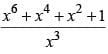is equal to       (SSC CHSL 2014)

Detailed Solution for SSC CGL Previous Year Questions: Algebra- 3 - Question 22

We have, x = 3 + 2√2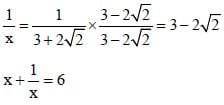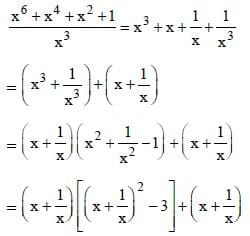= 6[62 – 3] + 6 = 198 + 6 = 204

SSC CGL Previous Year Questions: Algebra- 3 - Question 23

If x = p + (1/p) and y = p – (1/p), then value of x4 – 2x2 y2 + y4 is       (SSC CHSL 2014)

Detailed Solution for SSC CGL Previous Year Questions: Algebra- 3 - Question 23

x4 – 2x2y2 + y4 = (x2 – y2)2 = [(x + y)(x – y)]2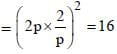SSC CGL Previous Year Questions: Algebra- 3 - Question 24

If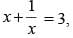then the value of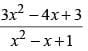is       (SSC CHSL 2014)

Detailed Solution for SSC CGL Previous Year Questions: Algebra- 3 - Question 24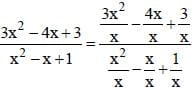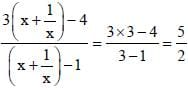SSC CGL Previous Year Questions: Algebra- 3 - Question 25

If a = 331, b = 336 and c = – 667, then the value of a3 + b3  + c3 – 3abc is        (SSC Sub. Ins. 2014)

Detailed Solution for SSC CGL Previous Year Questions: Algebra- 3 - Question 25

Here, a + b + c = 0
∴ a3 + b3 + c3 – 3abc = 0

SSC CGL Previous Year Questions: Algebra- 3 - Question 26

If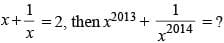(SSC Sub. Ins. 2014)

Detailed Solution for SSC CGL Previous Year Questions: Algebra- 3 - Question 26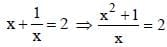x2 –2x + 1 = 0; (x – 1)2 = 0 ; x = 1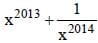= 1 + 1 = 2

SSC CGL Previous Year Questions: Algebra- 3 - Question 27

If p = 5/18 then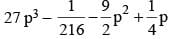is equal to       (SSC Sub. Ins. 2014)

Detailed Solution for SSC CGL Previous Year Questions: Algebra- 3 - Question 27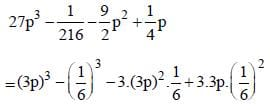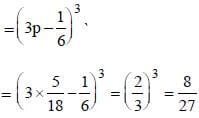SSC CGL Previous Year Questions: Algebra- 3 - Question 28

If a =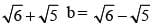then 2a2 – 5ab + 2b2 =       (SSC Sub. Ins. 2014)

Detailed Solution for SSC CGL Previous Year Questions: Algebra- 3 - Question 28

2a2 – 5ab + 2b2
2(a2 – 2ab + b2) – ab
2 (a – b)2 – ab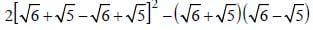2 × 4 × 5 – 1 = 39

SSC CGL Previous Year Questions: Algebra- 3 - Question 29

If x : y ::2 : 3 and 2 : x :: 4 : 8 the value of y is       (SSC Sub. Ins. 2014)

Detailed Solution for SSC CGL Previous Year Questions: Algebra- 3 - Question 29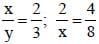x = 4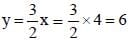SSC CGL Previous Year Questions: Algebra- 3 - Question 30

If x + y + z = 0, then the value of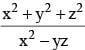is       (SSC CGL 2014)

Detailed Solution for SSC CGL Previous Year Questions: Algebra- 3 - Question 30

x + y + z = 0
y + z = –x
Squaring both sides
y2 + z2 + 2yz = x2
⇒ y2 + z2 = x2 – 2yz ...(1)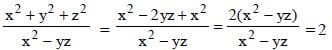SSC CGL Previous Year Questions: Algebra- 3 - Question 31

If a = 4.965, b = 2.343 and c = 2.622, then the value of a3 – b3 – c3 – 3abc is         (SSC CGL 2014)

Detailed Solution for SSC CGL Previous Year Questions: Algebra- 3 - Question 31

a = 4.965 ≈ 5, b = 2.343 ≈ 2
c = 2.622
a – b = c
taking cube both sides
a3 – b3 – 3a2b + 3ab2 = c3
a3 – b3 – c3 – 3ab(a – b) = 0
a3 – b3 – c3 – 3abc = 0

SSC CGL Previous Year Questions: Algebra- 3 - Question 32

If x = 2 + √3, then x2 + 1/(x2) is equal to       (SSC CGL 2014)

Detailed Solution for SSC CGL Previous Year Questions: Algebra- 3 - Question 32

x = 2 + √3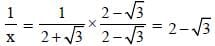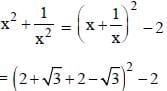= 16 – 2 = 14

SSC CGL Previous Year Questions: Algebra- 3 - Question 33

For what value of k, the system of  equations kx + 2y = 2 and 3x + y = 1 will be coincident?       (SSC CGL 2014)

Detailed Solution for SSC CGL Previous Year Questions: Algebra- 3 - Question 33

kx + 2y = 2 ...(1)
3x + y = 1 ...(2)
divide eqn (1) by (2)
(k/2) + y = 1
for system of equation to be coincident
k/2 = 3
k = 6

SSC CGL Previous Year Questions: Algebra- 3 - Question 34

The total area (in sq. unit) of the triangles formed by the graph of 4x + 5y = 40, x-axis, y-axis and x = 5 and y = 4 is       (SSC CGL 2014)

Detailed Solution for SSC CGL Previous Year Questions: Algebra- 3 - Question 34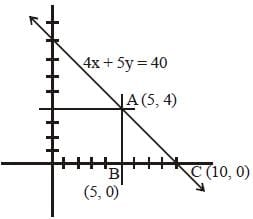Area of ΔABC = (1/2) × base × height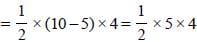Area = 10 sq unit.

SSC CGL Previous Year Questions: Algebra- 3 - Question 35

If a2 – 4a – 1 = 0,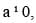then the value of a2 + 3a +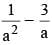is            (SSC CGL 2014)

Detailed Solution for SSC CGL Previous Year Questions: Algebra- 3 - Question 35

a2 – 4a – 1 = 0
a2 – 4a = 1
a (a – 4) = 1
a – 4 = (1/a)
a - (1/a) = 4 ...(1)
We have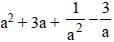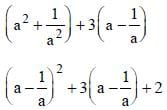42 + 3 × 4 + 2 = 30

SSC CGL Previous Year Questions: Algebra- 3 - Question 36

If a + b + c = 3, a+ b2 + c2 = 6 and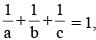where a, b, c are all non-zero, then 'abc' is equal to      (SSC CGL 2014)

Detailed Solution for SSC CGL Previous Year Questions: Algebra- 3 - Question 36

a + b + c = 3
Squaring both sides
a2 + b2 + c2 + 2 (ab + bc + ac) = 9
6 + 2 (ab + bc + ca) = 9
ab + bc + ca = 3/2 ...(1)
given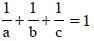⇒ ab + bc + ac = abc = 3/2 [from (1)]

SSC CGL Previous Year Questions: Algebra- 3 - Question 37

If a2 + b2 + c2 = 2a – 2b – 2, then the value of 3a – 2b + c is        (SSC CGL 2014)

Detailed Solution for SSC CGL Previous Year Questions: Algebra- 3 - Question 37

a2 + b2 + c2 = 2a – 2b – 2
(a2 – 2a + 1) + (b2 + 2b + 1) + c2 = 0
(a – 1)2 + (b + 1)2 + c2 = 0
This equation is possible if
a – 1 = 0, b + 1 = 0 and c = 0
a = 1, b = –1, c = 0
3a – 2b + c = 3 × 1 – 2 × (–1) + 0 = 3 + 2 = 5

SSC CGL Previous Year Questions: Algebra- 3 - Question 38

The straight line 2x + 3y = 12 passes through :         (SSC CGL 1st Sit. 2013)

Detailed Solution for SSC CGL Previous Year Questions: Algebra- 3 - Question 38

Putting y = 0 in 2x + 3y = 12, we get x = 6
Putting x = 0 in 2x + 3y = 12, we get, y = 4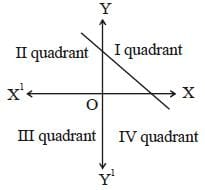SSC CGL Previous Year Questions: Algebra- 3 - Question 39

What number must be added to the expression 16a2 –12a to make it a perfect square?       (SSC CGL 1st Sit. 2013)

Detailed Solution for SSC CGL Previous Year Questions: Algebra- 3 - Question 39

a2 – 2ab + b2 = (a – b)2
∴ 16a2 – 12a
= (4a)2 – 2 × 4a × (3/2)
Hence, on adding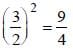SSC CGL Previous Year Questions: Algebra- 3 - Question 40

If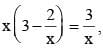then the value of x2 + 1/x2 is       (SSC CGL 1st Sit. 2013)

Detailed Solution for SSC CGL Previous Year Questions: Algebra- 3 - Question 40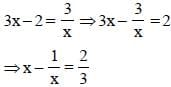On squaring both sides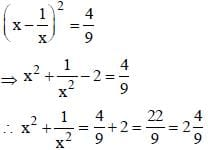## SSC CGL (Tier - 1) - Previous Year Papers (Topic Wise)

250 tests
Information about SSC CGL Previous Year Questions: Algebra- 3 Page
In this test you can find the Exam questions for SSC CGL Previous Year Questions: Algebra- 3 solved & explained in the simplest way possible. Besides giving Questions and answers for SSC CGL Previous Year Questions: Algebra- 3, EduRev gives you an ample number of Online tests for practice

250 tests